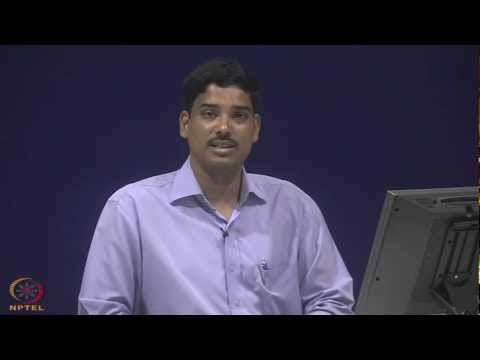Probability Methods in Civil EngineeringConcept of probability and statistics is very important to solve various civil engineering problems. In this video course, basic probability concept and different probabilistic models will be discussed to educate the audience about the vital role of such methods in civil engineering.
Concept and definition of random variables and different functions of random variables will be covered in the initial part of the course. Both univariate and multivariate functions will be discussed.
Concept of joint, marginal and conditional probability distributions, moments, expectations, correlation will also be discussed. Afterwards, focus is given to commonly used probability distribution functions in civil engineering.
Both discrete (binomial distribution, poisson’s distribution) and continuous distribution functions (normal, lognormal, exponential distribution, gamma distribution) will be discussed.
Concept of central limit theorem will also be also introduced. The course will be ended with the discussion of statistics and sampling.
In this part, goodness of fit tests, regression and correlation analyses, estimation of distribution parameters from statistics, hypothesis testing and their significance and Bayesian updating of distributions will be discussed.
Each topic is discussed with reference to different application problems and their solutions. The course is mainly designed for the post graduate students. However, general concept and basic applications may be helpful to under graduate students also.
 Sl.No. Topic No. of Hours 1. Introduction: Role of Probability in Civil Engineering Problems; Examples. 1 2. Random Events: Definition of basic random events; Application of set theory in definition of composite event operations; Probability of events and definition of probability axioms; Solution of real life examples from civil engineering. 6 3. Random Variables: Definition of random variables - discrete and continuous;Probability definitions - PMF, PDF, CDF; Moments and expectations. 6 4. Functions of Random Variables: Definition of probability distributions of functions of single random variables - exact methods and approximate methods; Moments and expectations of functions - direct and indirect methods. 6 5. Multiple Random Variables: Definition of joint, marginal, and conditional probability distributions; Definitions of moments and expectations, including the definition of correlation coefficient; Functions of multiple random variables. 6 6. Common Probability Models: Discrete random variables - binomial distribution, Poisson’s distribution; Continuous random variables - exponential distribution, gamma distribution; Central limit theorem; Normal and lognormal distributions; Extremal distributions. 8 7. Statistics and sampling: Goodness of fit tests; regression and correlation analyses; estimation of distribution parameters from statistics; Hypothesis testing and significance; Bayesian updating of distributions. 7